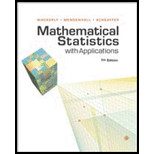## Solutions for Mathematical Statistics with Applications

### Book Details

1. What Is Statistics? Introduction. Characterizing a Set of Measurements: Graphical Methods. Characterizing a Set of Measurements: Numerical Methods. How Inferences Are Made. Theory and Reality. Summary.

2. Probability. Introduction. Probability and Inference. A Review of Set Notation. A Probabilistic Model for an Experiment: The Discrete Case. Calculating the Probability of an Event: The Sample-Point Method. Tools for Counting Sample Points. Conditional Probability and the Independence of Events. Two Laws of Probability. Calculating the Probability of an Event: The Event-Composition Methods. The Law of Total Probability and Bayes's Rule. Numerical Events and Random Variables. Random Sampling. Summary.

3. Discrete Random Variables and Their Probability Distributions. Basic Definition. The Probability Distribution for Discrete Random Variable. The Expected Value of Random Variable or a Function of Random Variable. The Binomial Probability Distribution. The Geometric Probability Distribution. The Negative Binomial Probability Distribution (Optional). The Hypergeometric Probability Distribution. Moments and Moment-Generating Functions. Probability-Generating Functions (Optional). Tchebysheff's Theorem. Summary.

4. Continuous Random Variables and Their Probability Distributions. Introduction. The Probability Distribution for Continuous Random Variable. The Expected Value for Continuous Random Variable. The Uniform Probability Distribution. The Normal Probability Distribution. The Gamma Probability Distribution. The Beta Probability Distribution. Some General Comments. Other Expected Values. Tchebysheff's Theorem. Expectations of Discontinuous Functions and Mixed Probability Distributions (Optional). Summary.

5. Multivariate Probability Distributions. Introduction. Bivariate and Multivariate Probability Distributions. Independent Random Variables. The Expected Value of a Function of Random Variables. Special Theorems. The Covariance of Two Random Variables. The Expected Value and Variance of Linear Functions of Random Variables. The Multinomial Probability Distribution. The Bivariate Normal Distribution (Optional). Conditional Expectations. Summary.

6. Functions of Random Variables. Introductions. Finding the Probability Distribution of a Function of Random Variables. The Method of Distribution Functions. The Methods of Transformations. Multivariable Transformations Using Jacobians. Order Statistics. Summary.

7. Sampling Distributions and the Central Limit Theorem. Introduction. Sampling Distributions Related to the Normal Distribution. The Central Limit Theorem. A Proof of the Central Limit Theorem (Optional). The Normal Approximation to the Binomial Distributions. Summary.

8. Estimation. Introduction. The Bias and Mean Square Error of Point Estimators. Some Common Unbiased Point Estimators. Evaluating the Goodness of Point Estimator. Confidence Intervals. Large-Sample Confidence Intervals Selecting the Sample Size. Small-Sample Confidence Intervals for u and u1-u2. Confidence Intervals for o2. Summary.

9. Properties of Point Estimators and Methods of Estimation. Introduction. Relative Efficiency. Consistency. Sufficiency. The Rao-Blackwell Theorem and Minimum-Variance Unbiased Estimation. The Method of Moments. The Method of Maximum Likelihood. Some Large-Sample Properties of MLEs (Optional). Summary.

10. Hypothesis Testing. Introduction. Elements of a Statistical Test. Common Large-Sample Tests. Calculating Type II Error Probabilities and Finding the Sample Size for the Z Test. Relationships Between Hypothesis Testing Procedures and Confidence Intervals. Another Way to Report the Results of a Statistical Test: Attained Significance Levels or p-Values. Some Comments on the Theory of Hypothesis Testing. Small-Sample Hypothesis Testing for u and u1-u2. Testing Hypotheses Concerning Variances. Power of Test and the Neyman-Pearson Lemma. Likelihood Ration Test. Summary.

11. Linear Models and Estimation by Least Sq

## More Editions of This Book

Corresponding editions of this textbook are also available below:

MATH.STATISTICS W/APPL.
5th Edition
ISBN: 9780534209162
Mathematical statistics with applications
6th Edition
ISBN: 9780534377410
Mathematical Statistics with Applications
7th Edition
ISBN: 9781111798789
Mathematical Statistics with Applications
7th Edition
ISBN: 9781133384380